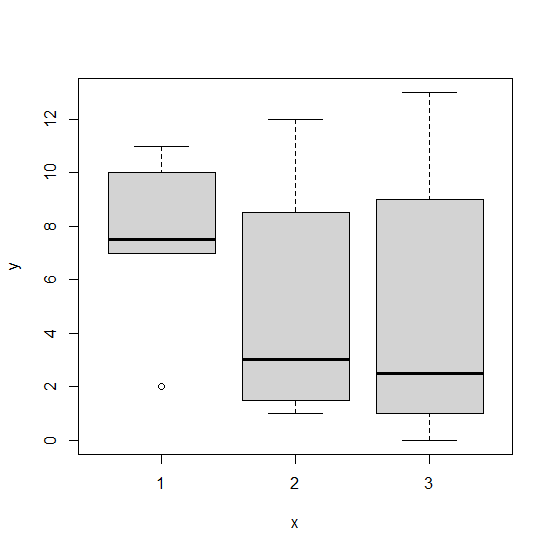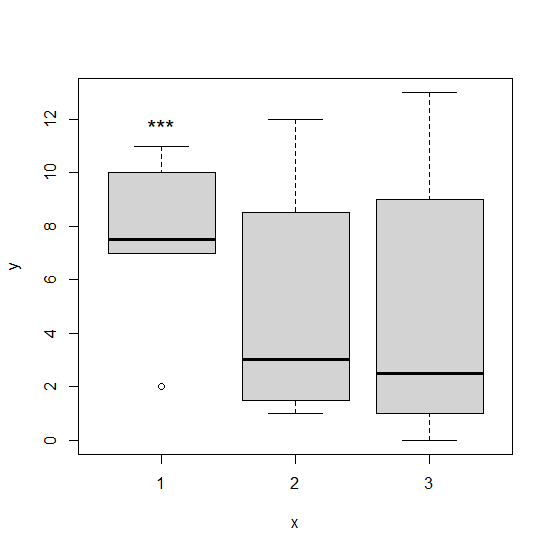# How to display star for significance in base R boxplot?

To display the star for significance in a base R boxplot, we can use text function. The text function will be helpful in defining the star sign (that is asterisk or *). If the significance is high then three stars are used and the significance is low then a single star is used. We need to use the appropriate position using x and y values.

## Example

Live Demo

Consider the below data frame −

x<−sample(1:3,20,replace=TRUE)
y<−c(rpois(10,10),rpois(10,2))
df<−data.frame(x,y)
df

## Output

x y
1 1 7
2 3 13
3 1 11
4 1 8
5 2 8
6 2 12
7 2 9
8 1 10
9 1 7
10 3 9
11 1 2
12 3 0
13 3 1
14 2 1
15 3 2
16 3 3
17 2 2
18 2 1
19 2 4
20 2 2

Creating the boxplot of y for categories in x −

## Example

boxplot(y~x)

## OutputAdding star to category 1 in the above plot −

## Example

text(x=1,y=max(df$y[df$x==1]),"***",pos=3,cex=1.5)

## Output